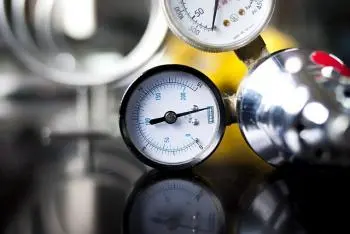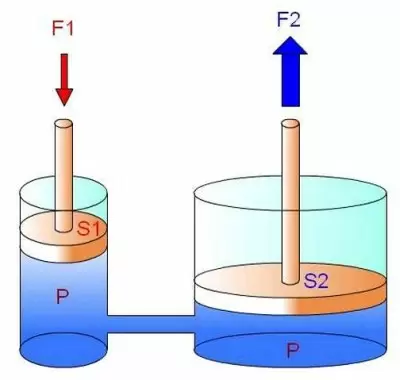# Pressure in Physics: Definition, Types, Units and PropertiesPressure is the physical magnitude that measures the force exerted on a unit area applied in a direction perpendicular to it. It can be calculated by dividing the applied force by the area over which that force is applied.

The mathematical formula to calculate pressure is:

Pressure = Force / Area

This physical magnitude can have different effects depending on the context in which it is applied. For example, in fluiddynamics and fluid mechanics in particular, pressure is related to properties such as density and height of the fluid. Many physical phenomena such as the buoyancy and operation of fluids in pipes and hydraulic systems depend on pressure.

In the field of nuclear power, the pressure in a nuclear reactor is important to ensure efficient heat transfer, control reactivity, maintain containment system integrity, ensure safety, and contribute to energy efficiency.

## Pressure Types

There are the following types:

• Hydrostatic pressure: that exerted by liquids that cannot be compressed on the objects that are in contact with them.

• Absolute pressure: it is the sum of the force per unit area of ​​a given system and that of the air that surrounds it.

• Gauge pressure (also called relative pressure) is the difference between absolute (true) and atmospheric.

• Atmospheric pressure: is the force per unit area that air exerts on the earth's surface. At sea level, 1 atm is equal to 101325 Pa.

• Arterial or blood: when we refer to the force exerted by blood on the inner surface of the arteries.

• Osmotic: is the force exerted per unit area of ​​a solution against a closed semipermeable membrane.

## Pressure Units

The unit of pressure in the International system of units is the pascal (Pa), in honor of Blaise Pascal. One pascal is the pressure exerted by a total force of one newton acting uniformly on one square meter (Pa = N / m2 ).

The Pascal (Pa) is a small unit, and sometimes it is convenient to use other units of measurement:

• Millimeter of mercury (mmHg): is a unit that is still used to measure pressure in medicine, meteorology, aviation and is equivalent to 133,322 387 415 Pa.

• bar. The use of this unit is accepted within the SI although it is not recommended. It is often used because it has a value very close to 1 atm. 1 bar = 100,000 Pa

• Hectopascals (HPa): This unit is used in meteorology and is equal to 100 Pa.

• Atmosphere (atm): The atmosphere is a unit that indicates the pressure that the earth's atmosphere produces on average on the earth's surface.

• Kiloponds per square centimeter (kp/cm2): This unit is used in engineering. The kilopond is equal to the weight of a mass of one kilogram (9.8 N).

• Pound of force per square inch (lbf/in²): it is a unit that belongs to the Anglo-Saxon system. To refer to it, the acronym psi (pounds-force per square inch) is also used.

## How Is Pressure Measured in Physics?

In physics, pressure is measured using a variety of instruments and techniques depending on the system or phenomenon being studied. Here are some examples:

1. Manometers: are used to measure the pressure in gases and liquids. These instruments measure pressure based on the deformation of a pressure sensitive element or by measuring the height of a column of liquid in equilibrium with the pressure.

2. Barometers: Barometers are instruments specifically designed to measure atmospheric pressure.

3. Pressure Sensors: In more advanced applications, electronic pressure sensors are used that convert pressure into an electrical signal.

4. U-tubes: One end of the tube is connected to the system where pressure is to be measured, and the other end is kept open or connected to a known reference point. The height difference of the fluids on the two sides of the U-tube provides a measure of the pressure difference.

## Pressure in Liquids, Solids and Gases

The pressure in liquids, solids and gases behaves differently due to the intrinsic properties of each state of matter as you can see below:

### Pressure in Liquids

In liquids, pressure is transmitted isotropically, that is, in all directions equally according to Pascal's principle.The pressure in a liquid depends on the depth at which it is found and the density of the liquid. At greater depth, the pressure increases due to the weight of the liquid above. This is due to hydrostatic pressure, which is the result of the force exerted by the weight of the liquid on a given area.

Hydrostatic pressure is calculated using the formula P = ρgh, where P is the pressure, ρ is the density of the liquid, g is the acceleration due to gravity, and h is the height or depth of the liquid.

### Pressure in Solids

In solids, pressure manifests itself as a force applied to a given surface. The pressure in a solid is the result of the distribution of force over the area of ​​contact.

As the applied force increases, the pressure also increases. Pressure in solids can be calculated by dividing the applied force by the area over which that force is applied.

### Pressure in Gases

In gases, the pressure is due to the collisions of the gas molecules against the walls of the container that contains it. The greater the number of molecules or the greater the kinetic energy of the molecules, there will be more collisions and, therefore, greater pressure.

The pressure of a gas can be calculated using the ideal gas law, which states that pressure is directly proportional to absolute temperature and the number of molecules present, and inversely proportional to volume.

This law is expressed mathematically as P = nRT/V, where P is the pressure, n is the number of moles of gas, R is the ideal gas constant, T is the absolute temperature, and V is the volume of the gas.

## The Pressure on People

In the context of health, pressure commonly refers to blood pressure, which is the force exerted by the blood against the walls of the arteries as the heart beats and relaxes. Blood pressure is expressed by two values: systolic pressure and diastolic pressure.

• Systolic pressure: It is the first value recorded when measuring blood pressure and represents the pressure in the arteries when the heart contracts and pumps blood to the body.

• Diastolic pressure: It is the second value recorded and represents the pressure in the arteries when the heart relaxes between beats.

Blood pressure is measured in millimeters of mercury (mmHg) and is expressed as a fraction, for example, 120/80 mmHg. The first number (the systolic pressure) is larger because it is measured during the contraction of the heart, while the second number (the diastolic pressure) is smaller because it is measured during the relaxation of the heart.

Author:

Published: November 18, 2021
Last review: July 16, 2023